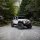Home Communities
IT Knowledge
Inspiration
Languages
EN

# MySQL - get size of every table in database (in MB)

0 points
Created by:martineau
450

In this article, we would like to show you how to get the size of each table in the database in MySQL.

Quick solution:

``````SELECT
TABLE_SCHEMA as `Database`,
TABLE_NAME AS `Table`,
ROUND(((data_length + index_length) / 1024 / 1024), 3) `Size (in MB)`
FROM INFORMATION_SCHEMA.TABLES
WHERE TABLE_SCHEMA = "your_database_name"
ORDER BY (data_length + index_length);``````

Note:

In the example above replace only `your_database_name`.

## Practical example

Let's say we have the following database:

What interests us will be the size of every table in the `dirask` database (`users` and `locations` table) shown in the image above. We want to get them with a query and display in descending order.

Query:

``````SELECT
TABLE_SCHEMA as `Database`,
TABLE_NAME AS `Table`,
ROUND(((data_length + index_length) / 1024 / 1024), 3) `Size (in MB)`
FROM INFORMATION_SCHEMA.TABLES
ORDER BY (data_length + index_length) DESC;``````

Result: